# How do you write a matrix multiplication in LaTeX?

Matrix multiplication is the complex LaTeX syntax. And take the help of multiple commands to form.

Before representing multiplication in a document, it is good to get acquainted with the following commands.

 \times × \cdots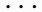\vdots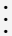\ddots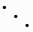\sum ∑

Which bracket the matrix will be bound to depend on the argument. In the case of matrix environment, five arguments have been defined for different brackets.

 pmatrix ( ) bmatrix [ ] Bmatrix { } vmatrix | | Vmatrix || ||

## m*n matrix multiplication in LaTeX

Arguments are passed according to different brackets in the matrix environment. Such as matrix, pmatrix, bmatrix etc.

And the elements of matrix below are bounded by square brackets, so the use of bmatrix argument has been passed in environment.

\documentclass{article}
\usepackage{amsmath}
\begin{document}

$\begin{bmatrix} a_{11} & a_{12} & \cdots & a_{1n}\\ a_{21} & a_{22} & \cdots & a_{2n}\\ \vdots & \vdots & \ddots & \vdots\\ a_{m1} & a_{m2} & \cdots & a_{mn} \end{bmatrix} \times \begin{bmatrix} b_{11} & b_{12} & \cdots & b_{1p}\\ b_{21} & b_{22} & \cdots & b_{2p}\\ \vdots & \vdots & \ddots & \vdots\\ b_{n1} & b_{n2} & \cdots & b_{np} \end{bmatrix} = \begin{bmatrix} c_{11} & c_{12} & \cdots & c_{1p}\\ c_{21} & c_{22} & \cdots & c_{2p}\\ \vdots & \vdots & \ddots & \vdots\\ c_{m1} & c_{m2} & \cdots & c_{mp} \end{bmatrix}$
$c_{ij}= a_{i1} b_{1j} + a_{i2} b_{2j} +\cdots+ a_{in} + b_{nj} = \sum_{k=1}^n a_{ik}b_{kj}$
\end{document}

Output :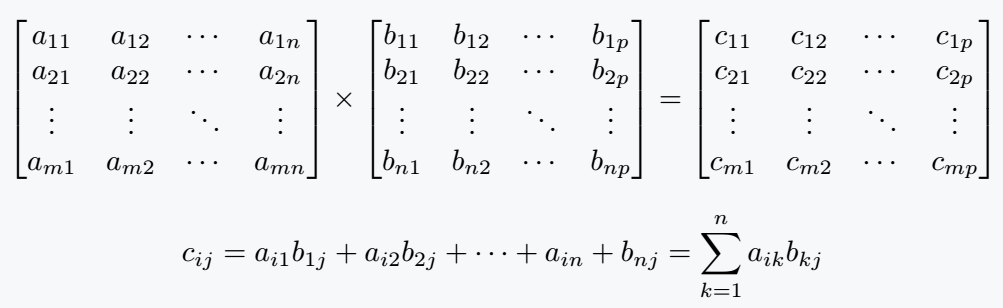And many of you want to know how 3 * 3 and 2 * 2 matrix multiplication is inserted in the document. It is discussed below.

### (i) 2*2 matrix multiplication

For the convenience of understanding this multiplication, you can take another step without doing a direct calculation. Which are in various books.

\documentclass{article}
\usepackage{amsmath}
\begin{document}

$\begin{bmatrix} 0 & 1\\ 0 & 0 \end{bmatrix} \times \begin{bmatrix} 0 & 0\\ 1 & 0 \end{bmatrix} = \begin{bmatrix} 1 & 0\\ 0 & 0 \end{bmatrix}$
\end{document}

Output :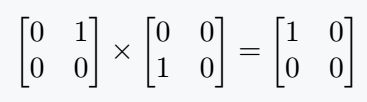### (ii) 3*3 matrix multiplication

You can use parenthesis instead of square brackets. For this, we need to use pmatrix argument instead of bmatrix.

\documentclass{article}
\usepackage{amsmath}
\begin{document}

$\begin{pmatrix} 2 & 7 & 3\\ 1 & 5 & 8\\ 0 & 4 & 1 \end{pmatrix} \times \begin{pmatrix} 3 & 0 & 1\\ 2 & 1 & 0\\ 1 & 2 & 4 \end{pmatrix} = \begin{pmatrix} 23 & 13 & 14\\ 21 & 21 & 33\\ 9 & 6 & 4 \end{pmatrix}$
\end{document}

Output :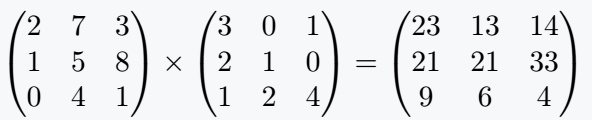## Vector matrix multiplication in LaTeX

Vector dot product is defined by the multiplication of row and column matrix.

\documentclass{article}
\usepackage{amsmath}
\begin{document}

$\begin{bmatrix} a_1 & a_2 & \cdots & a_n \end{bmatrix} \times \begin{bmatrix} b_1\\ b_2\\ \vdots\\ b_3 \end{bmatrix} =[a_1 \cdot b_1 + a_2 \cdot b_2 +\cdots+ a_n \cdot b_n]$
\end{document}

Output :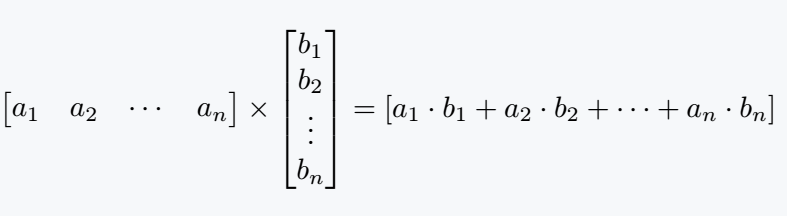#### Md Jidan Mondal

LaTeX expert with over 10 years of experience in document preparation and typesetting. Specializes in creating professional documents, reports, and presentations using LaTeX.